### Home > INT3 > Chapter 7 > Lesson 7.2.3 > Problem7-85

7-85.
1. Consider the functions f(x) =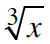and g(x) =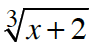. Homework Help ✎

1. Determine the point(s) of intersection by graphing.

2. Confirm the point of intersection by solving algebraically.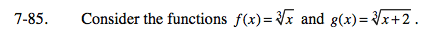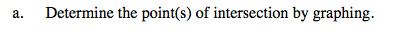The graph of g is the graph of f shifted 2 units to the left.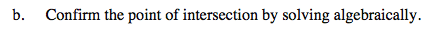Use the Equal Values Method (i.e., set the function equal to each other) to confirm that there is no solution.